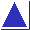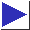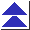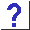# comp.lang.c FAQ list ·Question 20.9b

Q: How do I swap bytes?

A: V7 Unix had a swab function, but it seems to have been forgotten.

A problem with explicit byte-swapping code is that you have to decide whether to call it or not, based on the byte order of the data and the byte order of the machine in use. Question 20.9 shows how, but it's a nuisance.

A better solution is to define functions which convert between the known byte order of the data and the (unknown) byte order of the machine in use, and to arrange for these functions to be no-ops on those machines which already match the desired byte order. A set of such functions, introduced with the BSD networking code but now in wide use, is ntohs, htons, ntohl, and htonl. These are intended to convert between ``network'' and ``host'' byte orders, for ``short'' or ``long'' integers, where ``network'' order is always big-endian, and where ``short'' integers are always 16 bits and ``long'' integers are 32 bits. (This is not the C definition, of course, but it's compatible with the C definition; see question 1.1.) So if you know that the data you want to convert from or to is big-endian, you can use these functions. (The point is that you always call the functions, making your code much cleaner. Each function either swaps bytes if it has to, or does nothing. The decision to swap or not to swap gets made once, when the functions are implemented for a particular machine, rather than being made many times in many different calling programs.)

If you do have to write your own byte-swapping code, the two obvious approaches are again to use pointers or unions, as in question 20.9. Here is an example using pointers:

```void byteswap(char *ptr, int nwords)
{
char *p = ptr;
while(nwords-- > 0) {
char tmp = *p;
*p = *(p + 1);
*(p + 1) = tmp;
p += 2;
}
}
```

And here is one using unions:

```union word
{
short int word;
char halves;
};

void byteswap(char *ptr, int nwords)
{
register union word *wp = (union word *)ptr;
while(nwords-- > 0) {
char tmp = wp->halves;
wp->halves = wp->halves;
wp->halves = tmp;
wp++;
}
}
```

These functions swap two-byte quantities; the extension to four or more bytes should be obvious. The union-using code is imperfect in that it assumes that the passed-in pointer is word-aligned. It would also be possible to write functions accepting separate source and destination pointers, or accepting single words and returning the swapped values.

References: PCS Sec. 11 p. 179